Question

13.6 #3

The following mechanism for the gas phase decomposition of oxygen difluoride is consistent with the observed rate law.

.....step 1.....fast:......2 OF2 <-----> O2F4

.....step 2.....slow:....O2F4 -----> O2 + 2 F2

(1) What is the equation for the overall reaction? Use the smallest integer coefficients possible. If a box is not needed, leave it blank.
_____+_____----->_____+_____

 (2) Enter the formula of any species that acts as a reaction intermediate? If none leave box blank:

________

(3) Complete the rate law for the overall reaction that is consistent with this mechanism.
Use the form k[A]m[B]n... , where '1' is understood (so don't write it if it's a '1') for m, n etc.

 Rate =

_________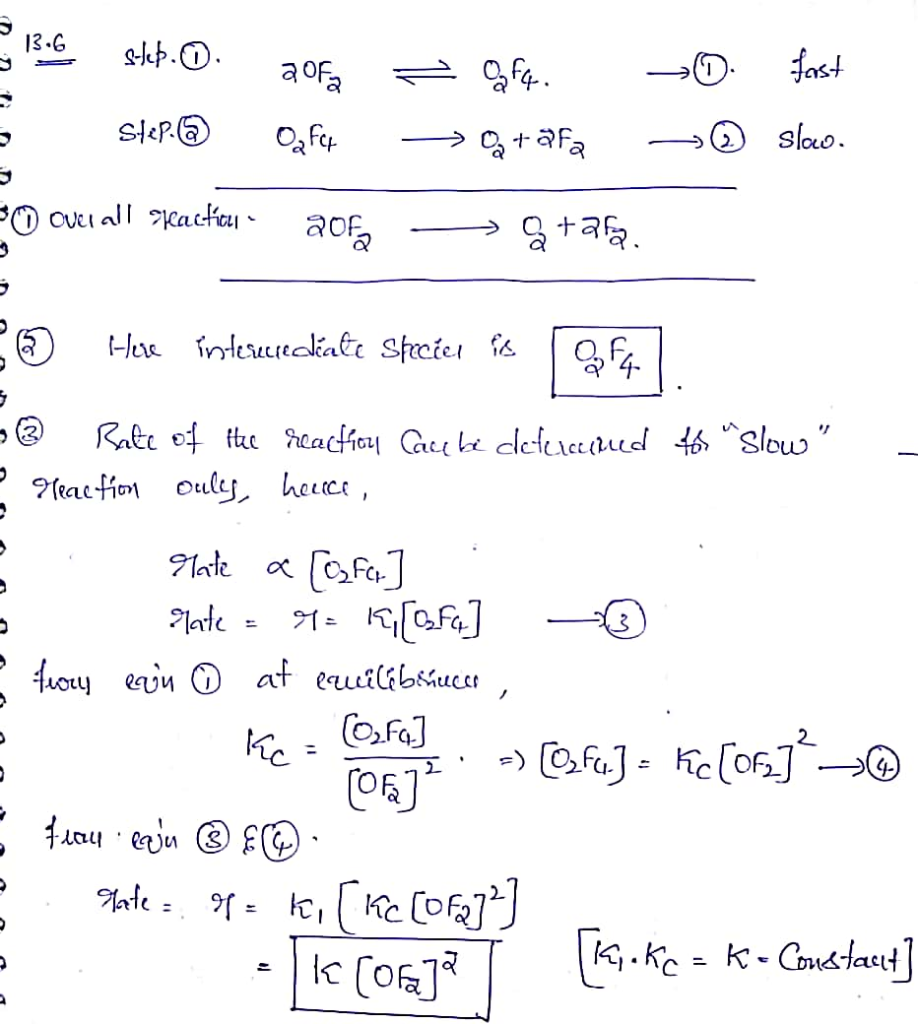#### Earn Coins

Coins can be redeemed for fabulous gifts.

Similar Homework Help Questions
• ### A mechanism for the gas phase reaction of fluorine with chlorine dioxide that is consistent with...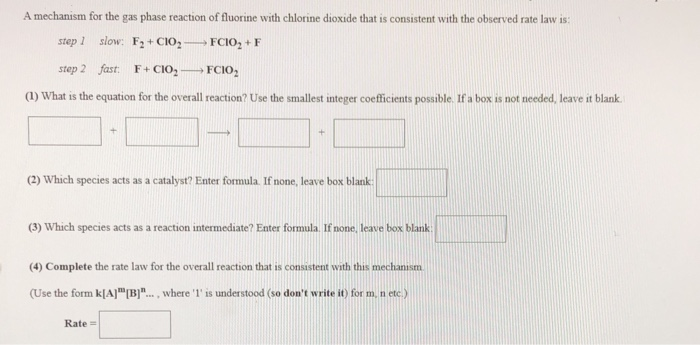A mechanism for the gas phase reaction of fluorine with chlorine dioxide that is consistent with the observed rate law is: step slow: F2+CIO, FCIO, + F step 2 fast: F+CIO, FCIO, (1) What is the equation for the overall reaction? Use the smallest integer coefficients possible. If a box is not needed, leave it blank. (2) Which species acts as a catalyst? Enter formula. If none, leave box blank (3) Which species acts as a reaction intermediate? Enter formula....

• ### The following is a proposed mechanism for the gas phase decomposition of nitryl chloride. step 1...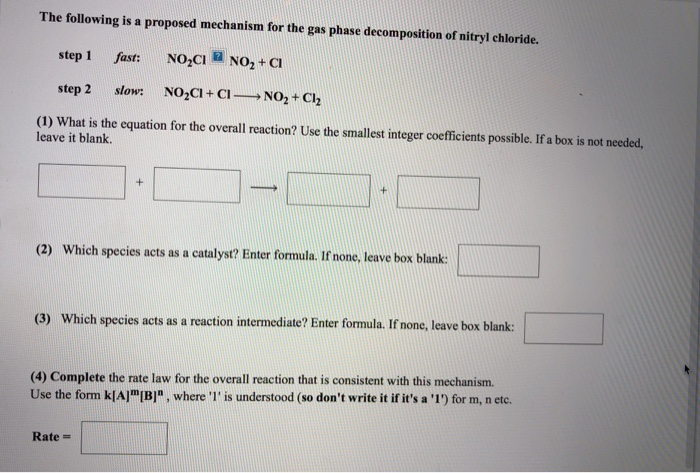The following is a proposed mechanism for the gas phase decomposition of nitryl chloride. step 1 fast: NO2CNO2+CI step 2 slow: NO2CI+CI NO2 + Cl2 (1) What is the equation for the overall reaction? Use the smallest integer coefficients possible. If a box is not needed, leave it blank. (2) Which species acts as a catalyst? Enter formula. If none, leave box blank: (3) Which species acts as a reaction intermediate? Enter formula. If none, leave box blank: (4) Complete...

• ### A mechanism for the gas phase reaction of fluorine with chlorine dioxide that is consistent with...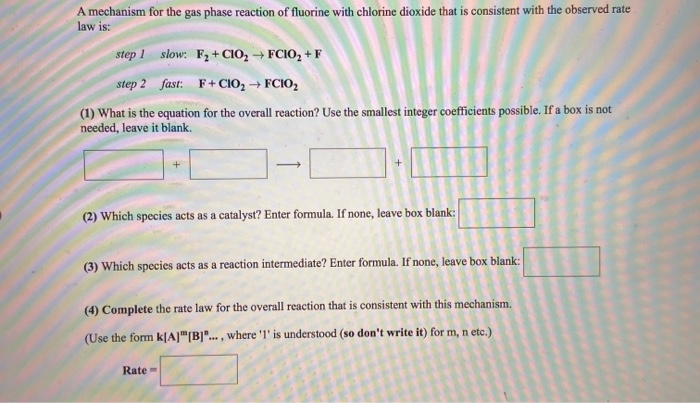A mechanism for the gas phase reaction of fluorine with chlorine dioxide that is consistent with the observed rate law is: step 1 slow: Fz+CIO, step 2 fast: F+CIO, FCIO, +F FCIO, (1) What is the equation for the overall reaction? Use the smallest integer coefficients possible. If a box is not needed, leave it blank. (2) Which species acts as a catalyst? Enter formula. If none, leave box blank: (3) Which species acts as a reaction intermediate? Enter formula....

• ### A student proposed the following mechanism for the gas phase reaction of fluorine with chlorine dioxide....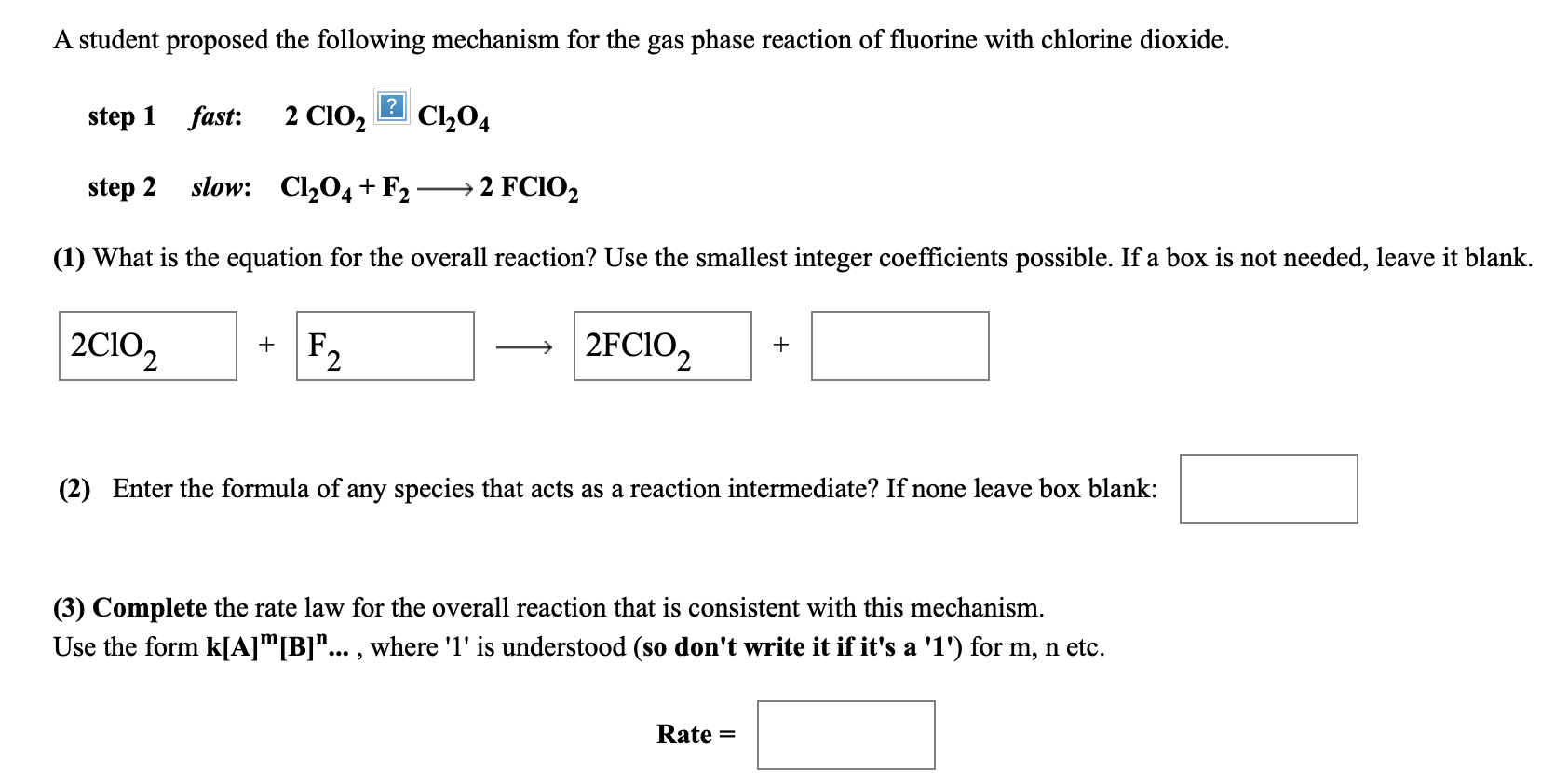A student proposed the following mechanism for the gas phase reaction of fluorine with chlorine dioxide. step 1 fast: 2 CIO, 2 C104 step 2 slow: C1204 +F2 ——2 FCIO2 (1) What is the equation for the overall reaction? Use the smallest integer coefficients possible. If a box is not needed, leave it blank. 20102 + F2 - 2FC10, + (2) Enter the formula of any species that acts as a reaction intermediate? If none leave box blank: (3) Complete...

• ### A proposed mechanism for the gas phase reaction between nitrogen monoxide and oxygen is as follows:...A proposed mechanism for the gas phase reaction between nitrogen monoxide and oxygen is as follows: ..... step 1 ..... fast: ...... NO + O2NO3 ..... step 2 ..... slow: .... NO3 + NO 2 NO2 (1) What is the equation for the overall reaction? Use the smallest integer coefficients possible. If a box is not needed, leave it blank. +      + (2) Which species acts as a catalyst? Enter formula. If none, leave box blank: (3) Which species...

• ### A student proposed the following mechanism for the gas phase reaction of fluorine with chlorine dioxide....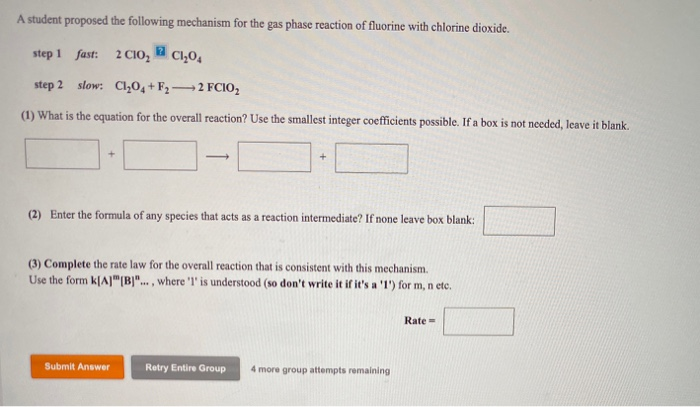A student proposed the following mechanism for the gas phase reaction of fluorine with chlorine dioxide. step 1 fast: 2C10, C1,04 step 2 slow: Cl,04 + F2 — 2 FCIO2 (1) What is the equation for the overall reaction? Use the smallest integer coefficients possible. If a box is not needed, leave it blank. (2) Enter the formula of any species that acts as a reaction intermediate? If none leave box blank: (3) Complete the rate law for the overall...

• ### The following mechanism has been proposed for the reaction between nitrogen monoxide and oxygen in the...The following mechanism has been proposed for the reaction between nitrogen monoxide and oxygen in the gas phase. .....step 1.....fast:......2 NO ---> N2O2 .....step 2.....slow:....N2O2 + O2 ----> 2 NO2 (1) What is the equation for the overall reaction? Use the smallest integer coefficients possible. If a box is not needed, leave it blank. _______ +    ________     _________ + ________ (2)    Enter the formula of any species that acts as a reaction intermediate? If none leave box...

• ### The following mechanism has been proposed for the conversion of ozone to oxygen in the gas...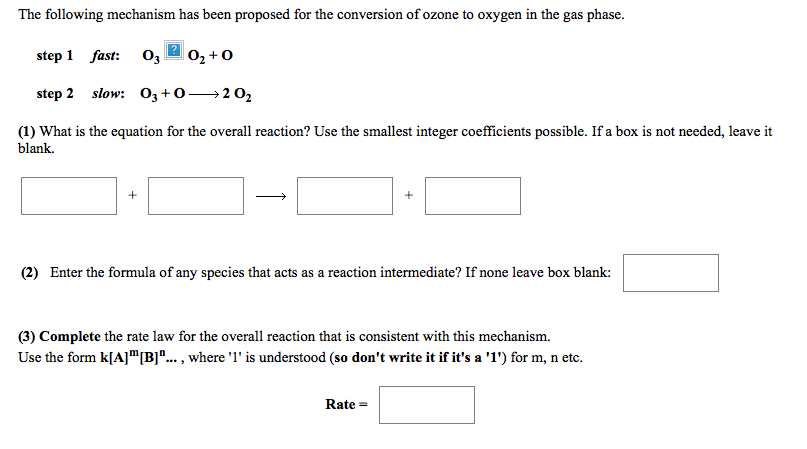The following mechanism has been proposed for the conversion of ozone to oxygen in the gas phase. step 1 fast: O3O step 2 slow: 03 + 0-202 (1) What is the equation for the overall reaction? Use the smallest integer coefficients possible. If a box is not needed, leave it blank. (2) Enter the formula of any species that acts as a reaction intermediate? If none leave box blank: (3) Complete the rate law for the overall reaction that is...

• ### please i need help with all these questions.. thanks so much Use the References to access...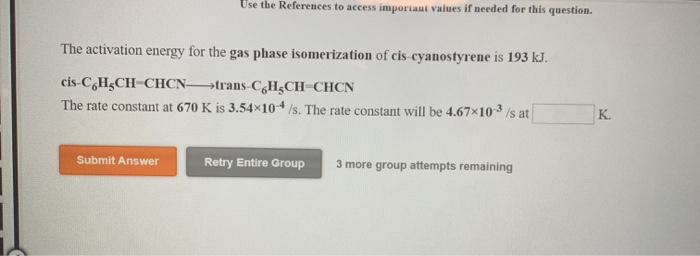please i need help with all these questions.. thanks so much Use the References to access important values if needed for this question. The activation energy for the gas phase isomerization of cis cyanostyrene is 193 kJ. cis-CH CH CHCN-trans-C H CH CHCN The rate constant at 670 K is 3.54x10-4 /s. The rate constant will be 4.67x10 3 /s at Submit Answer Retry Entire Group 3 more group attempts remaining (References] Use the References to access important values if...

• ### Ozone decomposes to molecular oxygen: 2 O3(g) ⇋ 3 O2(g). The following is a proposed mechanism...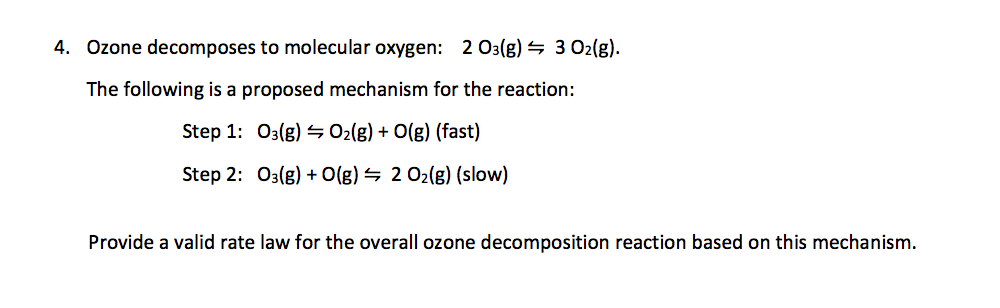Ozone decomposes to molecular oxygen: 2 O3(g) ⇋ 3 O2(g). The following is a proposed mechanism for the reaction: Step 1: O3(g) ⇋ O2(g) + O(g) (fast) Step 2: O3(g) + O(g) ⇋ 2 O2(g) (slow) Provide a valid rate law for the overall ozone decomposition reaction based on this mechanism. 4. Ozone decomposes to molecular oxygen: 203(g) — 3 O2(g). The following is a proposed mechanism for the reaction: Step 1: 03(g) – O2(g) + O(g) (fast) Step 2:...